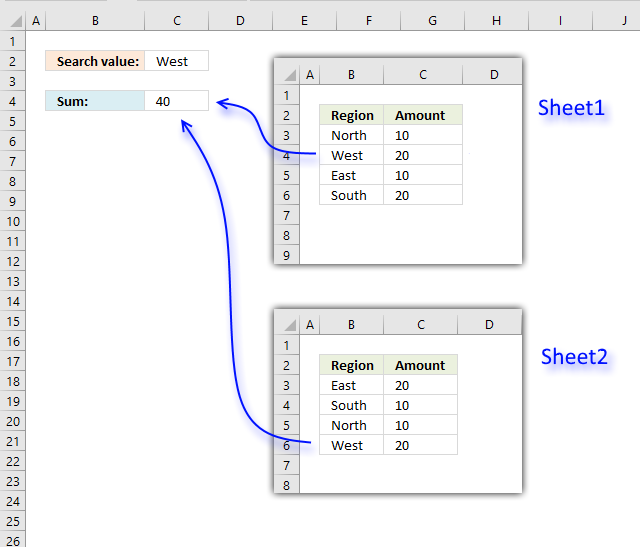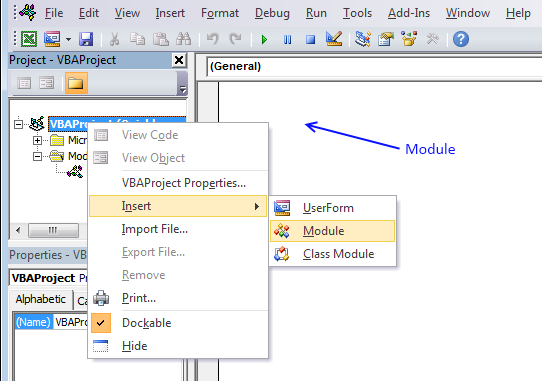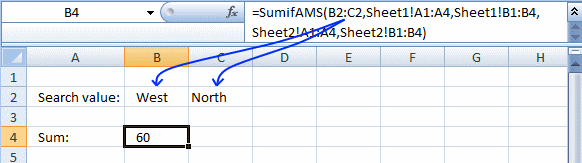Author: Oscar Cronquist Article last updated on January 28, 2019This post describes a User Defined Function that searches multiple ranges and adds corresponding values across worksheets in a workbook.

A User defined function is a custom function that anyone can build and use, you simply copy the code below and paste it to a code module in your workbook

This custom function works like the SUMIF function except that you can use multiple lookup and sum ranges.

Formula in cell C4:

=SUMIFAMS(C2, Sheet1!B3:B6, Sheet1!C3:C6, Sheet2!B3:B6, Sheet2!C3:C6)

### User Defined Function Syntax

SUMIFAMS(lookup_value, lookup_range, sum_range)

### Arguments

 Parameter Text lookup_value Required. The value(s) you want to look for. lookup_range Required. The range you want to search. sum_range Required. The range you want to add. [lookup_range_2] Optional. You may have up to 127 additional argument pairs. [sum_range_2] Optional.

### VBA code

```'Name function and arguments
Function SUMIFAMS(lookup_value As Range, ParamArray cellranges() As Variant)
'Declare variables and data types
Dim i As Integer, rng1 As Variant, temp As Single, a As Boolean
Dim rng2 As Variant, value As Variant, j As Single

'Make sure that the number of cell ranges are an even number. There must be a lookup_range and a sum_range.
If (UBound(cellranges) + 1) Mod 2 <> 0 Then
'Display a message box.
MsgBox "The number of range arguments must be even. 2, 4 , 8 ... and so on"
'End function so the user can correct the problem.
Exit Function
End If
'Iterate through all cell ranges
For i = LBound(cellranges) To UBound(cellranges) Step 2
'Check that the number of rows in both cell ranges match
If cellranges(i).Rows.Count <> cellranges(i + 1).Rows.Count Then
MsgBox "The number of rows in range arguments don't match."
End If
'Make sure that the ranges only contain one column
If cellranges(i).Columns.Count <> 1 Then
MsgBox "Range arguments can only have size one column each."
Exit Function
End If
'Save ranges to an array variable
rng1 = cellranges(i).value
rng2 = cellranges(i + 1).value
'Iterate through values in array variable
For j = LBound(rng1) To UBound(rng1)
'Iterate through each lookup value if there are more than one
For Each value In lookup_value
'Make a comparison (not case sensitive)
If UCase(rng1(j, 1)) = UCase(value) Then a = True
Next value
'Add corresponding number to temp variable if a= True
If a = True Then temp = temp + rng2(j, 1)
a = False
Next j
Next i
'Return total to worksheet
SumifAMS = temp
End Function

```

### Where to copy vba code?1. Press Alt-F11 to open visual basic editor
2. Click Module on the Insert menu
3. Copy and paste vba code.
4. Exit visual basic editor

You can also use multiple search values, the returned number is a total based on both search values.### Download Excel file through email

Enter your email address to receive the workbook. Note, an email will actually be sent to you.
* You will also get a weekly newsletter, unsubscribe anytime!